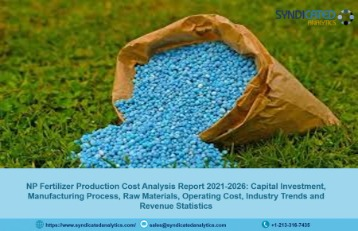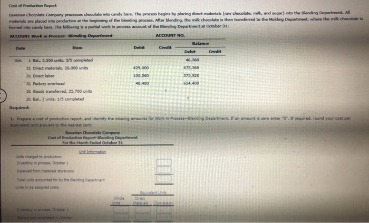# Production Cost Report ExplainedA separate cost of production report is prepared for each processing department. The cost of ending inventory in a Process Costing system is equal to the sum of all costs for units transferred out plus labor and factory overhead costs, less any materials that were released but not yet used in production. The cost of goods sold is the sum of all costs for units started and completed during the period. The Jessica Company produces a product used to clean mirrors and head lights of vehicles. The company uses a process costing and has two processing departments – department X and department Y.

In the weighted average method, we divided total costs by total Equivalent Units, but here is where the FIFO method is significantly different. Unfinished units (work-in-process) in this department have to be converted to Equivalent Units. In this case, since materials are all added at the beginning, the Equivalent Units for Direct Materials is 100% of the actual units, but the Equivalent Units for the Conversion Costs allocation is 25% of actual units or 250 EUs. Units started during the period, finished, and then transferred out are 100% complete, so the Equivalent Units and the actual physical units are the same. This is where equivalent units are different than the normal formula, but only for beginning inventory.

## 5 Preparing a Production Cost Report

Remaining units were in process estimated to be 50%, 40%, 60% completed as to materials, labor and factory overhead respectively. Costs of materials, labor and overhead were Rs. 49,875, Rs. 59,925 and Rs. 40,032 respectively. A work-in-process inventory (wip) is an account that represents the cost of partially completed products. In Process Costing, the wip inventory accumulates the costs of all units that were started and completed during the period, but had not been transferred out of the department as of the end of the accounting period. From the accounting records, we see that total direct materials transferred to the mixing department in February were \$3,575 and that direct labor and manufacturing overhead totaled \$3,640.

If the beginning work-in-process inventory is 10% done, then the factor to use to calculate EUs to finish it up this month is 90%. The MST Manufacturing Company produces one product that passes through a single process in a manufacturing cycle lasting approximately 18 days. Our writing and editorial staff are a team of experts holding advanced financial designations and have written for most major financial media publications. Our work has been directly cited by organizations including Entrepreneur, Business Insider, Investopedia, Forbes, CNBC, and many others.

## Production Cost Report – Explained

Job-order costing is used when there is production of a variety of products or for one time jobs. This system records costs at the time they are incurred and it is easier to have control over the job. With 750 started in January, the other 2,250 were started and completed in February. Some costs will not change at all with a change in sales volume (e.g., monthly rent for the production facility). To predict what will happen to profit in the future, we must understand how costs behave with changes in the number of units sold (sales volume).

Therefore, there will generally be some work-in-process inventories at the end of each month.Direct materials Rs. 19,400, direct labor Rs. 24,250 and factory overhead Rs. 4,850 was charged to production. Under weighted average method, it is done by dividing the total of beginning inventory cost and cost added during the period by the equivalent units of production computed under weighted average method. Click here to read how equivalent units of production are computed using weighted average method.

## Cost of Production Report (CPR) Questions and Answers FAQs

The 750 shells in production at the end of January were 60% complete as to conversion costs and 100% complete as to direct materials, so in February they will need 40% more conversion but 0% more direct materials. The mixing department started another 3,250 shells during February, and at the end of the month, there were still 1,000 shells being mixed and prepped for baking. They were only 25% complete as to conversion but 100% of the direct materials had been added (because they are added at the beginning of the process). Because we calculated EUs based on completed units, including EUs that represent the effort it took to complete the beginning inventory, we divide ONLY costs added during the period by our Equivalent Units. Notice that the basic data are at the top of the spreadsheet, and the rest of the report is driven by formulas.

The processing starts in department X where three different chemicals are mixed together in equal proportions. After mixing in department X, the partially completed units are transferred to department Y. We have 4,000 total units for which to account, with 750 in process at the beginning of the month, and the last batch that is still in process at the end of the month will be 1,000 shells once it is done. On the last day of February, it was only 25% through the process, meaning that the EUs for ending inventory for direct materials was 1,000 units and for conversion costs was 25% of 1,000 units which is 250 EUs. A production cost report identifies the total cost (direct materials, labor, and overhead), of producing a product. We started with 750 units that were 100% complete as to materials and 60% processed, so the beginning work-in-process EUs for direct materials is 0% of 750 and for conversion costs is 40% of 750 which is 300.

• Other names used for cost of production report are production cost report and production report.
• Remaining units were in process estimated to be 50%, 40%, 60% completed as to materials, labor and factory overhead respectively.
• Notice that the basic data are at the top of the spreadsheet, and the rest of the report is driven by formulas.
• They were only 25% complete as to conversion but 100% of the direct materials had been added (because they are added at the beginning of the process).

Establish the total inventory in production by adding units started into production to beginning work in process (what was left only partially finished at the end of the prior month). The beginning inventory of 750 plus the 3,250 pie shells worth of materials placed into production during the month gives us 4,000 total units to account for. 10,000 units were started in process out of which 9,400 units were transferred to next department and remaining 600 units were 1/2 complete as to materials, labor and overhead.

## Cost of production report (CPR) – weighted average method

This section summarizes the flow of physical units through the relevant processing department and shows the equivalent units for materials and conversion costs. The percentage of completion of any units in work in process beginning and ending inventory is also shown in this section. The quantity schedule also guides in preparing the other two sections of the cost of production report. In order to arrive at cost per unit of output, total of each cost element is divided by the number of units produced. For this purpose, where at the end of costing period, there are some partially completed units in process, these units must be stated in terms of equivalent completed units.

## What are the main reasons of using a job-order costing system?

Under the weighted average method, costs transferred in from last month are added to this month’s costs and distributed across all units. This is the simplest way to account for beginning inventory costs, but not always the most accurate. For example, if costs are going up, the cost of those 750 pie shells in beginning work in process inventory would be less than the cost of the 1,000 pie shells in ending inventory. According to the accounting records, direct materials transferred to the mixing department were costed at \$3,575 and direct labor and factory overhead were \$3,640.

Process Costing always follows the same process while job-order costing applies to each job separately. A Total costs to be accounted for (step 2) must equal total costs accounted for (step 4). EUs for beginning inventory is the complement of last month’s ending inventory because now you are finishing them up.

Each month, the data at the top are changed to reflect the current month’s activity, and the production cost report takes care of itself. Other names used for cost of production report are production cost report and production report. Now, check your understanding of the FIFO method of computing ending and work-in-process inventory using process costing. In circumstances where the product is flowing in batches like this, FIFO gives us a more accurate per unit cost for both beginning and ending work-in-process inventory. Multiply the cost per equivalent unit by the number of equivalent units for each category of units accounted for.

These equivalent units are added to units completed by the department to arrive at equivalent production. Then total cost is divided by this equivalent production figure to calculate unit cost. The cost of production report (CPR) is a document used in process costing system that summarizes information about the flow of units and costs through the work in process account of a processing department.